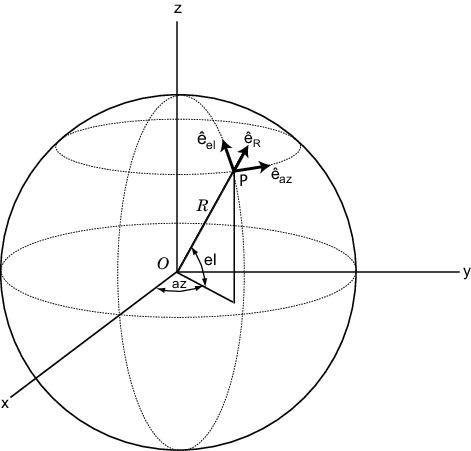# cart2sphvec

Convert vector from Cartesian components to spherical representation

Since R2020a

## Syntax

``vs = cart2sphvec(vr,az,el)``

## Description

example

````vs = cart2sphvec(vr,az,el)` converts the components of a vector or set of vectors, `vr`, from their representation in a local Cartesian coordinate system to a spherical basis representation contained in `vs`. A spherical basis representation is the set of components of a vector projected into a basis given by $\left({\stackrel{^}{e}}_{az},{\stackrel{^}{e}}_{el},{\stackrel{^}{e}}_{R}\right)$. The orientation of a spherical basis depends upon its location on the sphere as determined by azimuth, `az`, and elevation, `el`.```

## Examples

collapse all

Start with a vector in Cartesian coordinates pointing along the z-direction and located at 45° azimuth, 45° elevation. Compute its components with respect to the spherical basis at that point.

```vr = [0;0;1]; vs = cart2sphvec(vr,45,45)```
```vs = 3×1 0 0.7071 0.7071 ```

## Input Arguments

collapse all

Vector in Cartesian basis representation specified as a 3-by-1 column vector or 3-by-N matrix. Each column of `vr` contains the three components of a vector in the right-handed Cartesian basis x,y,x.

Example: `[4.0; -3.5; 6.3]`

Data Types: `double`
Complex Number Support: Yes

Azimuth angle specified as a scalar in the closed range [–180,180]. Angle units are in degrees. To define the azimuth angle of a point on a sphere, construct a vector from the origin to the point. The azimuth angle is the angle in the xy-plane from the positive x-axis to the vector's orthogonal projection into the xy-plane. As examples, zero azimuth angle and zero elevation angle specify a point on the x-axis while an azimuth angle of 90° and an elevation angle of zero specify a point on the y-axis.

Example: `45`

Data Types: `double`

Elevation angle specified as a scalar in the closed range [–90,90]. Angle units are in degrees. To define the elevation of a point on the sphere, construct a vector from the origin to the point. The elevation angle is the angle from its orthogonal projection into the xy-plane to the vector itself. As examples, zero elevation angle defines the equator of the sphere and ±90° elevation define the north and south poles, respectively.

Example: `30`

Data Types: `double`

## Output Arguments

collapse all

Spherical representation of a vector returned as a 3-by-1 column vector or 3-by-N matrix having the same dimensions as `vs`. Each column of `vs` contains the three components of the vector in the right-handed $\left({\stackrel{^}{e}}_{az},{\stackrel{^}{e}}_{el},{\stackrel{^}{e}}_{R}\right)$ basis.

collapse all

### Spherical basis representation of vectors

Spherical basis vectors are a local set of basis vectors which point along the radial and angular directions at any point in space.

The spherical basis is a set of three mutually orthogonal unit vectors $\left({\stackrel{^}{e}}_{az},{\stackrel{^}{e}}_{el},{\stackrel{^}{e}}_{R}\right)$ defined at a point on the sphere. The first unit vector points along lines of azimuth at constant radius and elevation. The second points along the lines of elevation at constant azimuth and radius. Both are tangent to the surface of the sphere. The third unit vector points radially outward.

The orientation of the basis changes from point to point on the sphere but is independent of R so as you move out along the radius, the basis orientation stays the same. The following figure illustrates the orientation of the spherical basis vectors as a function of azimuth and elevation:For any point on the sphere specified by az and el, the basis vectors are given by:

Any vector can be written in terms of components in this basis as $v={v}_{az}{\stackrel{^}{e}}_{az}+{v}_{el}{\stackrel{^}{e}}_{el}+{v}_{R}{\stackrel{^}{e}}_{R}$. The transformations between spherical basis components and Cartesian components take the form

`$\left[\begin{array}{c}{v}_{x}\\ {v}_{y}\\ {v}_{z}\end{array}\right]=\left[\begin{array}{ccc}-\mathrm{sin}\left(az\right)& -\mathrm{sin}\left(el\right)\mathrm{cos}\left(az\right)& \mathrm{cos}\left(el\right)\mathrm{cos}\left(az\right)\\ \mathrm{cos}\left(az\right)& -\mathrm{sin}\left(el\right)\mathrm{sin}\left(az\right)& \mathrm{cos}\left(el\right)\mathrm{sin}\left(az\right)\\ 0& \mathrm{cos}\left(el\right)& \mathrm{sin}\left(el\right)\end{array}\right]\left[\begin{array}{c}{v}_{az}\\ {v}_{el}\\ {v}_{R}\end{array}\right]$`

.

and

$\left[\begin{array}{c}{v}_{az}\\ {v}_{el}\\ {v}_{R}\end{array}\right]=\left[\begin{array}{ccc}-\mathrm{sin}\left(az\right)& \mathrm{cos}\left(az\right)& 0\\ -\mathrm{sin}\left(el\right)\mathrm{cos}\left(az\right)& -\mathrm{sin}\left(el\right)\mathrm{sin}\left(az\right)& \mathrm{cos}\left(el\right)\\ \mathrm{cos}\left(el\right)\mathrm{cos}\left(az\right)& \mathrm{cos}\left(el\right)\mathrm{sin}\left(az\right)& \mathrm{sin}\left(el\right)\end{array}\right]\left[\begin{array}{c}{v}_{x}\\ {v}_{y}\\ {v}_{z}\end{array}\right]$.

## Version History

Introduced in R2020a